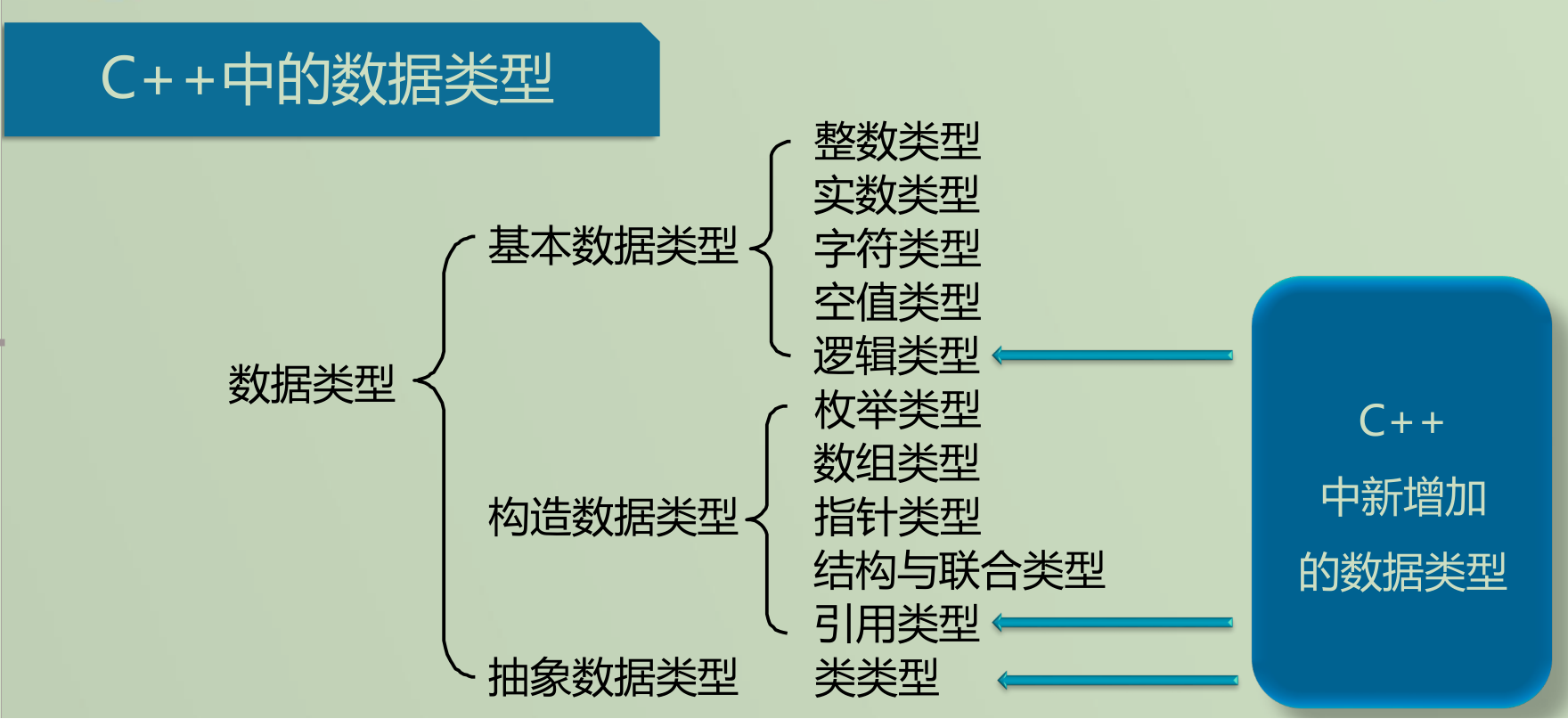【C++从入门到入土】第一篇：从C到C++

2021/8/21 19:41:45 浏览：

文章目录

• 前言
• 一、C++是什么？
• 二、C++基础
• 1、输入输出特点和用法
• 2、数据类型与表达式
• C++中的数据类型
• 基本数据类型
• 构造数据类型
• 3、动态内存管理
• 用new运算符实现动态内存分配
• 用delete运算符释放动态分配的内存
• 4、函数重载
• 函数的缺省参数
• 5、内联函数
• 6、引 用
• 引用作为函数的返回值
• 7、常 量
• 总结

一、C++是什么？

• C++是C语言的继承，它既可以进行C语言的过程化程序设计，又可以进行以抽象数据类型为特点的基于对象的程序设计，还可以进行以继承和多态为特点的面向对象的程序设计。

• C++擅长面向对象程序设计的同时，还可以进行基于过程的程序设计，因而C++就适应的问题规模而论，大小由之。

• C++不仅拥有计算机高效运行的实用性特征，同时还致力于提高大规模程序的编程质量与程序设计语言的问题描述能力

二、C++基础

1、输入输出特点和用法

1. 无输入输出语句，而是使用输入输出库，istream和ostream
2. 系统中的预定义流对象cin和cout：
输入流：cin处理标准输入，即键盘输入；
输出流：cout处理标准输出，即屏幕输出。
3. 流：从某种IO设备上读入或写出的字符序列
4. 使用cin、cout这两个流对象时需要：#include
5. 标准库定义了4个IO对象：

cin:istream对象，标准输入
cout:ostream对象，标准输出
cerr:标准错误。用来输出警告和错误信息给程序的使用者
clog:用于产生程序执行的一般信息

1. “<<”是预定义的插入符，作用在cout上可实现屏幕输出，格式如下：

cout<<表达式<<表达式<<……

1. 不同类型可以在一个语句输出！
2. ">>”是预定义的提取符，作用在cin上可实现键盘输入，格式如下：

cin>>表达式>>表达式>.……

//输入2个整数求和
#include<iostream>
int main()
{
std::cout<<“Enter_two_numbers”<<std::endl;
intv1,v2;
std::cin>>v1>>v2;
std::cout<<“the_sum_of”<<v1<<and<<
<<“is”<<v1+v2<<std::endl;
return0;
}

2、数据类型与表达式

C++中的数据类型构造数据类型

{
char name;
bool sexy;
};

p};

class Clock
{
private:
int hour,minute,second;
public:

}

3、动态内存管理

用new运算符实现动态内存分配

• 第一种用法，分配一个变量:
P = new T;
T是任意类型名，P是类型为T *的指针。
动态分配出一片大小为sizeof(T)字节的内存空间，并且将该
内存空间的起始地址赋值给P。比如：
int *p;
p = new int;
*p = 6;
• 第二种用法,分配一个数组：
P = new T[N];
T : 任意类型名
P : 类型为T *的指针
N : 要分配的数组元素的个数，可以是整型表达式 动态分配出一片大小为sizeof(T)*N字节的内存空间，并且将该内存空间的起始地址赋值给P。
动态分配数组示例：
int *p;
int i = 5;
p = new int[i * 20];
p = 10;
p = 30;//编译没问题。运行时导致数组越界

用delete运算符释放动态分配的内存

• 用“new”动态分配的内存空间，一定要用“delete”运算符进行释放
delete 指针；//该指针必须指向new出来的空间
int * p = new int;
* p = 5;
delete p;
delete p; //导致异常，一片空间不能被delete多次
• 用“delete”释放动态分配的数组，要加“[]” delete [ ] 指针；//该指针必须指向new出来的数组
int * p = new int;
p = 1;
delete [ ] p;

A)char * p = new int;
p = ‘a’;
delete p;
B) int *p = new int;
p = 100;
delete p;
C) char * p = new char;
p = ‘K’;
delete [] p;

B选项释放数组忘记在数组名前加"[]"

4、函数重载

(1) int Max(double f1,double f2) { }
(2) int Max(int n1,int n2) { }
(3) int Max(int n1,int n2,int n3) { }
Max(3.4,2.5); //调用 (1)
Max(2,4); //调用 (2)
Max(1,2,3); //调用 (3)
Max(3,2.4); //error,二义性

函数的缺省参数

C++中，定义函数的时候可以让最右边的连续若干个参

void func( int x1, int x2 = 2, int x3 = 3)
{ }
func(10 ) ; //等效于 func(10,2,3)
func(10,8) ; //等效于 func(10,8,3)
func(10, , 8) ; //不行,只能最右边的连续若干个参数缺省
• 函数参数可缺省的目的在于提高程序的可扩充性。
• 即如果某个写好的函数要添加新的参数，而原先那些调用该函数的语句，未必需要使用新增的参数，那么为了避免对原先那些函数调用语句的修改，就可以使用缺省参数。
• 默认形参值必须按照从右向左的顺序声明。在有默认值的形参右边，不能出现无默认值的形参。
• 在相同的作用域内，默认形参值的说明应保持唯一；但在不同的作用域内，允许说明不同的默认形参值。

5、内联函数

#define abs(a) ((a) < 0 ?(a):(a))
int main()
{
int m = -2, ret=abs(++m); abs(++m);
ret=((++m) < 0 ?(++m) : (++m))
return 0;
}
• 最后ret的值为是0。问题出在编译器在遇到宏时只是进行简单的
宏替换。
• 宏的好处是没有类似于普通函数调用时的系统开销， 并且宏定义的参数可以适宜大多数类型的数据。
• 宏定义也有缺点：有时会产生不可预料的副作用

C++中的内联函数既具有宏定义的优点，又克服了宏定义的缺点。

• 函数调用是有时间开销的。如果函数本身只有几条语句，执行非常快，而且函数被反复执行很多次，相比之下调用函数所产生的这个开销就会显得比较大。
• 为了减少函数调用的开销，引入了内联函数机制。编译器处理对内联函数的调用语句时，是将整个函数的代码插入到调用语句处，而不会产生调用函数的语句。
• 定义内联函数使用关键字inline。
inline int Max(int a,int b)
{
if( a > b) return a;
return b;
}

• 并非所有函数都需要定义为内联函数，一般只会将那 些频繁被调用的，并且函数体较小的（只有几条语句） 函数定义为内联函数。
• 内联函数内不允许有循环语句和switch语句，否则按 照普通函数来处理。

A) 多个重载函数的参数个数必须不同。
B) 两个函数，参数表相同，返回值类型不同，它们是重载关系。
C) 调用一个第二个和第三个参数都有有缺省值的函数时，可以不写第二个实参而写第三个实参。
D) 使用内联函数的目的是提高程序的运行速度。

6、引 用

int n = 4;
int & r = n; // r引用了 n, r的类型是 int &

• 某个变量的引用，等价于这个变量，相当于该变量的一个别名。
• 定义引用时一定要将其初始化成引用某个变量。
• 初始化后，它就一直引用该变量，不会再引用别的变量了。
• 引用只能引用变量，不能引用常量和表达式。

C语言中，如何编写交换两个整型变量值的函数?

void swap( int * a, int * b)
{
int tmp;
tmp = * a; * a = * b; * b = tmp;
}
int n1, n2;
swap(& n1,& n2) ; // n1,n2

void swap( int & a, int & b)
{
int tmp;
tmp = a; a = b; b = tmp;
}
int n1, n2;
swap(n1,n2) ; // n1,n2的值被交换

引用作为函数的返回值

int n = 4;
int & SetValue() { return n; }
int main()
{
SetValue() = 40;
cout << n;
return 0;
}

7、常 量

1. 可读性差 ；
2. 可维护性差 ；

C++为符号常量提供了const方法，格式为：

const 数据类型 符号常量名 = 数值；

• 用“＃define”定义的符号常量只在编译时完成宏替换（简单的字 符串替换），在程序运行期间不占内存空间。
• 用“const”定义的符号常量在程序运行期间占据内存空间，只是 用const来指明该内存空间的只读约束。

const int MAX_VAL = 23 ；
const string SCHOOL_NAME = “Peking University” ；

• 不可通过常量指针修改其指向的内容
int n,m;
const int * p = & n;
p = 5; // 编译出错
n = 4; //ok
p = &m; //ok, 常量指针的指向可以变化
• 不能把常量指针赋值给非常量指针，但反过来可以
const int * p1; int * p2;
p1 = p2; //ok
p2 = p1; //error
p2 = (int * ) p1; //ok, 强制类型转换
• 函数参数为常量指针时，可避免函数内部不小心改变参数指针所指地方的内容
void MyPrintf( const char * p )
{
strcpy( p,"this"); // 编译出错
printf("%s",p); //ok
}

int n;
const int & r = n;
r 的类型是 const int &

int n;
const int & r = n;
r = 5; //error
n = 4; //ok

const T & 和T & 是不同的类型!!!
T & 类型的引用或T类型的变量可以用来初始化
const T & 类型的引用。
const T 类型的常变量和const T & 类型的引用则